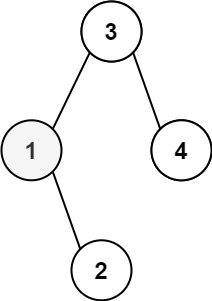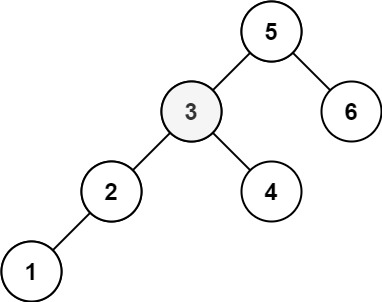# 230. Kth Smallest Element in a BST

Given the `root` of a binary search tree, and an integer `k`, return the `kth` smallest value (1-indexed) of all the values of the nodes in the tree.

Example 1:```Input: root = [3,1,4,null,2], k = 1
Output: 1
```

Example 2:```Input: root = [5,3,6,2,4,null,null,1], k = 3
Output: 3
```

Constraints:

• The number of nodes in the tree is `n`.
• `1 <= k <= n <= 104`
• `0 <= Node.val <= 104`

## The solution to the above question is-

/**
* Definition for a binary tree node.
* struct TreeNode {
*     int val;
*     TreeNode *left;
*     TreeNode *right;
*     TreeNode() : val(0), left(nullptr), right(nullptr) {}
*     TreeNode(int x) : val(x), left(nullptr), right(nullptr) {}
*     TreeNode(int x, TreeNode *left, TreeNode *right) : val(x), left(left), right(right) {}
* };
*/
class Solution {
public:
int n=0;
int ans=0;
void inorder(TreeNode* root,int k){
if(root==NULL)return;

inorder(root->left,k);
n++;
if(n==k)ans=root->val;
inorder(root->right,k);
}

int kthSmallest(TreeNode* root, int k) {

inorder(root,k);
return ans;
}
};